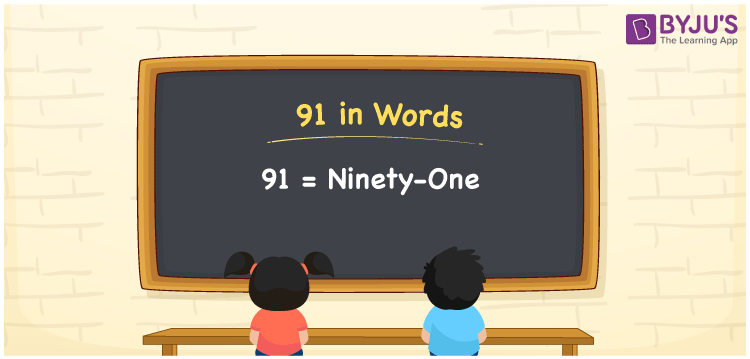# 91 in Words

91 in words can be written as Ninety-one. In this article, you will learn in brief about the concept of counting and its applications in our daily lives. For example, if you buy 1 kg of rice for Rs. 91 at the grocery store, then you can say that “I bought 1 kg of rice for Ninety-one Rupees at the grocery store”. Here numbers in words are written with the help of the English alphabet. So, 91 can be read as “Ninety-one” in words.

 91 in words Ninety-one Ninety-one in Numbers 91

## 91 in English Words## How to Write 91 in Words?

The place value chart for 91 is discussed here in a table form. The number 91 has two digits. The place value of each digit can be understood easily here.

 Tens Ones 9 1

The expanded form of 91 is given here:

9 × Ten + 1 × One

= 9 × 10 + 1 × 1

= 90 + 1

= 91

= Ninety-one

Hence, 91 in words is written as Ninety-one.

91 is a natural number that precedes 92 and succeeds 90.

91 in words – Ninety-one

Is 91 an odd number? – Yes

Is 91 an even number? – No

Is 91 a perfect square number? – No

Is 91 a perfect cube number? – No

Is 91 a prime number? – No

Is 91 a composite number? – Yes

## Frequently Asked Questions on 91 in Words

Q1

### How should you write 91 in words?

91 can be written as “Ninety-one” in words.
Q2

### What is the value of 95 minus 4?

The value of 95 minus 4 is 91. Therefore, 91 in words is Ninety-one.
Q3

### Is 91 an even number?

No, 91 is not an even number as it is not completely divisible by 2.